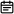# 674. 最长连续递增序列2019-06-06

## 题目链接

https://leetcode-cn.com/problems/longest-continuous-increasing-subsequence/

## 题目描述

``````输入: [1,3,5,4,7]

``````输入: [2,2,2,2,2]

## 解题方案

### 思路

• 标签：遍历
• 过程：

• count为当前元素峰值，ans为最大峰值
• 初始化count = 1
• 从0位置开始遍历，遍历时根据前后元素状态判断是否递增，递增则count++，递减则count=1
• 如果count>ans，则更新ans
• 直到循环结束
• 时间复杂度：O(N)

### 代码

``````class Solution {
public int findLengthOfLCIS(int[] nums) {
if(nums.length <= 1)
return nums.length;
int ans = 1;
int count = 1;
for(int i=0;i<nums.length-1;i++) {
if(nums[i+1] > nums[i]) {
count++;
} else {
count = 1;
}
ans = count > ans ? count : ans;
}
return ans;
}
}``````

### 画解